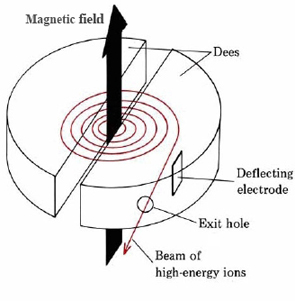# 19.5: Bombardment with Positive Ions

$$\newcommand{\vecs}{\overset { \rightharpoonup} {\mathbf{#1}} }$$ $$\newcommand{\vecd}{\overset{-\!-\!\rightharpoonup}{\vphantom{a}\smash {#1}}}$$$$\newcommand{\id}{\mathrm{id}}$$ $$\newcommand{\Span}{\mathrm{span}}$$ $$\newcommand{\kernel}{\mathrm{null}\,}$$ $$\newcommand{\range}{\mathrm{range}\,}$$ $$\newcommand{\RealPart}{\mathrm{Re}}$$ $$\newcommand{\ImaginaryPart}{\mathrm{Im}}$$ $$\newcommand{\Argument}{\mathrm{Arg}}$$ $$\newcommand{\norm}{\| #1 \|}$$ $$\newcommand{\inner}{\langle #1, #2 \rangle}$$ $$\newcommand{\Span}{\mathrm{span}}$$ $$\newcommand{\id}{\mathrm{id}}$$ $$\newcommand{\Span}{\mathrm{span}}$$ $$\newcommand{\kernel}{\mathrm{null}\,}$$ $$\newcommand{\range}{\mathrm{range}\,}$$ $$\newcommand{\RealPart}{\mathrm{Re}}$$ $$\newcommand{\ImaginaryPart}{\mathrm{Im}}$$ $$\newcommand{\Argument}{\mathrm{Arg}}$$ $$\newcommand{\norm}{\| #1 \|}$$ $$\newcommand{\inner}{\langle #1, #2 \rangle}$$ $$\newcommand{\Span}{\mathrm{span}}$$

A powerful method of artificially inducing nuclear reactions is the bombardment of a sample of matter with ions. When the bombarding particle is positively charged, which is usually the case, it must have a very high kinetic energy to overcome the coulombic repulsion of the nucleus being bombarded. This is particularly necessary if the nucleus has a high nuclear charge. To give these charged particles the necessary energy, an accelerator or “atom smasher” such as a cyclotron must be used. The cyclotron was developed mainly by E. O. Lawrence (1901 to 1958) at the University of California. A schematic diagram of a cyclotron is shown in Figure $$\PageIndex{1}$$. Two hollow D-shaped plates (dees) are enclosed in an evacuated chamber between the poles of a powerful electromagnet. The two dees are connected to a source of high-frequency alternating current, so that when one is positive, the other is negative. Ions are introduced at the center and are accelerated because of their alternate attraction to the left- and right-hand dees. Since the magnetic field would make ions traveling at constant speed move in a circle, the net result is that they follow a spiral path as they accelerate until they finally emerge at the outer edge of one of the dees.Figure $$\PageIndex{1}$$: A cyclotron. The spiral path of the ions is shown in color.

Some examples of the kinds of nuclear reactions which are possible with the aid of an accelerator are as follows:

${}_{\text{12}}^{\text{24}}\text{Mg + }{}_{\text{1}}^{\text{1}}\text{H}\to \text{ }{}_{\text{11}}^{\text{21}}\text{Na + }{}_{\text{2}}^{\text{4}}\text{He } \nonumber$

${}_{\text{3}}^{\text{7}}\text{Li + }{}_{\text{1}}^{\text{2}}\text{H}\to \text{ }{}_{\text{4}}^{\text{8}}\text{Be + }{}_{\text{0}}^{\text{1}}n\text{ } \nonumber$

${}_{\text{46}}^{\text{106}}\text{Pb + }{}_{\text{2}}^{\text{4}}\text{He +}\to \text{ }{}_{\text{47}}^{\text{109}}\text{Ag + }{}_{\text{1}}^{\text{1}}\text{H} \nonumber$

A particularly interesting type of nuclear reaction performed in an accelerator is the production of the transuranium elements. These elements have atomic numbers greater than that of uranium (92) and are too unstable to persist for long in nature. The heaviest of them can be prepared by bombarding nuclei which are already heavy with some of the lighter nuclei:

${}_{\text{92}}^{\text{238}}\text{U + }{}_{\text{6}}^{\text{12}}\text{C}\to \text{ }{}_{\text{98}}^{\text{246}}\text{Cf + 4}{}_{\text{0}}^{\text{1}}n \nonumber$

${}_{\text{92}}^{\text{238}}\text{U + }{}_{\text{7}}^{\text{14}}\text{N}\to \text{ }{}_{\text{99}}^{\text{247}}\text{Es + 5}{}_{\text{0}}^{\text{1}}n\text{ } \nonumber$

${}_{\text{98}}^{\text{252}}\text{Cf + }{}_{\text{5}}^{\text{10}}\text{B}\to \text{ }{}_{\text{103}}^{\text{257}}\text{Lr + 5}{}_{\text{0}}^{\text{1}}n\text{ } \nonumber$

This page titled 19.5: Bombardment with Positive Ions is shared under a CC BY-NC-SA 4.0 license and was authored, remixed, and/or curated by Ed Vitz, John W. Moore, Justin Shorb, Xavier Prat-Resina, Tim Wendorff, & Adam Hahn.